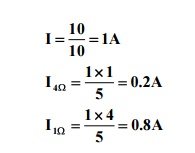Home | | Circuit Theory | Important Short Questions and Answers: Network Reduction and Network Theorems for DC And AC Circuits

# Important Short Questions and Answers: Network Reduction and Network Theorems for DC And AC Circuits

Electrical and electronics - Network Reduction and Network Theorems for DC And AC Circuits - Important Short Questions and Answers: Network Reduction and Network Theorems for DC And AC Circuits

1.                 State   Theveninâ€™s. theorem

Any complex network consisting of linear, bilateral and lumped elements can be replaced by a simple circuit consisting of single voltage source in series with resistance. It is also called as Helmholtzâ€™s. theorem

2.                 State   Nortonâ€™s.  theorem

Any complex network consisting of linear, bilateral and lumped elements can be replaced by a simple circuit consisting of single current source in parallel with resistance.

3.                 State   Superposition   theorem.

In a linear, bilateral and lumped circuit elements that is energized by two or more sources, the response(current through or voltage across) in any resistor is equal to individual responses in it, when sources acts separately.

4.       State Maximum power transfer theorem.

It states that the maximum power transferred to the load occurs when the load resistance is equal to the source resistance (equivalent resistance).

Condition for maximum power transfer RL = RTH

5.       State Reciprocity theorem.

It states that in a linear, bilateral, single source circuit, the ratio of excitation to response is constant when the position of excitation and response are interchanged.

6.Draw   the   Theveninâ€™s   equivalent   circuit.7. Draw the Nortonâ€™s   equivalent   circuit8.                 What   are   the   applications   of   Theveninâ€™s   theorem?

1. To find the particular branch current in an electrical network while the resistance of that

branch is varied with all other elements of the network remaining unchanged.

2. Used in sensitivity analysis.

9.                 What   are   the   advantages   of   Theveninâ€™s   theorem?

1. Applicable to circuits containing any type of load â€“linear or non linear or time varying.

2. Applicable to circuits with load containing sources.

3. Applicable to circuits with load having initial conditions on passive

elements.

10.What   are   the   limitations   of   Theveninâ€™s theorem?

1. Load should be connected to network containing linear elements only.

2. There should be no controlled source or magnetic coupling with the elements of load.

11.What are the applications of Maximum power transfer theorem? (AU-MAY08)

1. It is used for impedance matching.

2. It is used in communication circuits, where the power or circuit currents are low.

12.What are the limitations of Reciprocity theorem?

1. Only one source is present in the network.

2. Initial conditions should be zero.

3. The network is linear.

4. Impedance matrix is symmetric.

5. Dependent sources present in the network, even if they are linear, are excluded.

13.under what conditions, the super position theorem may be applied to the circuit.

It is applicable to all time variant linear networks. It holds good for all possible locations, types and waveforms of the independent systems. The theorem applies both in time domain and frequency domain.

15. Write short notes about superposition theorem.

It is valid only for linear circuits. It is not valid for power responses. When the superposition theorem is applied to any circuit. the dependent voltage source in the circuit is always active.

16.       State   under   what   circumstances   Theveninâ€™s

Theveninâ€™s equivalent circuit is useful in particular branch current in a network as the resistance of that branch is varied while all other

resistances and sources remain constant.

17. What is meant by short and open circuits?

A short is conductor with zero resistance and an open is an infinitely large resistance. When we short circuit the resistance we replace the resistance by a conductor with zero resistance and when we open the circuit a resistance we remove the resistance.

18. Find the current through 10 â„¦ resistor in the following circuit.19. Determine the current through 1 â„¦  and 4 â„¦ resistors.20. Find the current I in the circuit.

When 2A source is acting alone.When both sources are acting together I=2A

21. Find the theveninâ€™s   equivalent for   the   circuit   shown.22.Find   the   Nortonâ€™s   equivalent.   for   the   circuit shown.23. Find the value of RL for maximum power transfer.

Condition for maximum power transfer RL=RTH24. What is the property of additivity and homogeneity?

The principle of super position is a combination of additivity and homogeneity.

The property of addivity says that the response in a circuit due to number of sources is given by sum of response due to individual sources acting alone.

The property of homogeneity says that if al the sources are multiplied by a constant, then the response is also multiplied by the same constant.

25. By using superposition theorem, find the current through the ammeter.

Response due to 10V source I1

Response due to 5V source I 2

Total response = IStudy Material, Lecturing Notes, Assignment, Reference, Wiki description explanation, brief detail
Electrical and electronics : Circuit Theory : Network Reduction and Network Theorems for DC And AC Circuits : Important Short Questions and Answers: Network Reduction and Network Theorems for DC And AC Circuits |